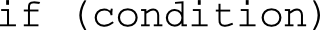# 5.1 - if 陳述

C++ 最簡單的選擇結構 (selection structure) 為單一個 if 陳述，在關鍵字 if 後接一個小括弧，該小括弧內的運算式即為條件。形式如下``````#include <iostream>

int main() {
char a = 'h';
char b = 'h';

if (a == b) {
std::cout << "Welcome to my world!" << std::endl;
}

return 0;
}

/* 《程式語言教學誌》的範例程式
http://kaiching.org/
檔名：u0501_1.cpp
功能：示範 C++ 的 if 陳述
作者：張凱慶*/``````

 \$ g++ u0501_1.cpp \$ ./a.out Welcome to my world! \$

``if (a == b) {``

``````#include <iostream>
int main() {
char a = 'h';
char b = 'k';

if (a == b) {
std::cout << "Welcome to my world!" << std::endl;
}

if (a != b) {
std::cout << "Free your mind." << std::endl;
}

return 0;
}

/* 《程式語言教學誌》的範例程式
http://kaiching.org/
檔名：u0501_2.cpp
功能：示範 C++ 的 if 陳述
作者：張凱慶*/``````

 \$ g++ u0501_2.cpp \$ ./a.out Free your mind. \$